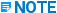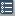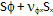# International Code Stresses - CAESAR II - Installation & Upgrade

## CAESAR II Quick Reference

Language
English (United States)
Product
CAESAR II
Search by Category
CAESAR II Version
12

The international code-based stress equations and load case labels used by CAESAR II for actual and allowable stresses are shown below.

The load case recommendations made by CAESAR II are usually sufficient for code compliance. CAESAR II does not recommend occasional load cases. Occasional loads are unknown in origin and you must specify them.

Code Equation

Allowable

Stoomwezen

Slp + 0.75iMa / Z

< f

SUS

iMC / Z

< fe

EXP

Slp + 0.75i(Ma + Mb) / Z

< 1.2f

OCC

CODETI

Sl = Slp + 0.75iMA / Z

< Sh

SUS

iMC / Z

< f[1.25Sc + 0.25Sh]Eh/Ec

EXP

Slp + 0.75iMA / Z + 0.75iMB / Z

< kSh

OCC

Alternate Method:

Config switch set to TRUE.

Sl = Slp + Fax/Am + Sb

< Sh

SUS

(Sb2 + 4St2)1/2

< f[1.25(Sl + Sh) – Sl

EXP

Slp + Fax/Am + Sb

< kSh

OCC

Sb = {[(iiMi)2 + (ioMo)2]1/2} / ZClick Configureor Tools > Configure/Setup to select the method.

Fully restrained pipe (FAC = 1.0)

Sh - SLThis only applies if SL is negative (compression)

Where:

S = specified minimum yield strength

T = temperature factor (Table 4.4)

Sh = PDo/2tcor hoop stress

Where:
P = design pressure
Do = outside diameter of pipe
tcor = corroded pipe wall thickness

< 0.9ST

OPE

SL = nSh - ECa(T2-T1) longitudinal stress

Where:
n = Poisson’s ratio
EC = elastic modulus at ambient temperature
a = thermal expansion coefficient
T2-T1 = temperature range between cold and hot conditions

Buried pipe with soil restraints modeled (FAC = 0.001)

Sh – SL + SB

Where:

SB = iMb/Z bending stress

Where:
i = stress intensification factor
Mb = resultant bending moment
Z = (p/64)(Do4-Di4)/0.5Do section modulus
Di = inside pipe diameter (nominal)

< ST

OPE

Fully above ground (FAC = 0.0)

|Slp+Fax/Am|+SB+Sh

Where:

Slp = PDi2/(Do2 – Di2) longitudinal pressure stress

Fax = axial force

Am = metal cross-sectional area

< ST

OPE

SE = (SB2 + 4St2)1/2

Where:
St = Mt/2Z torsion stress
Mt is the torsion moment

< 0.72ST

EXP

0.5Sh + Sb

< SFLT

OCC

Where:

Sb = bending stress due to sustained and occasional loading combined

F = design factor (0.800)

L = location factor (Table 4.2)

Canadian Z662 Chapter 11

sh = (Pi – Pe)Do/2tmin

< SyFAT

OPE, SUS, OCC

Seq = (sh2 + Sl2 - shSl + 3t2)1/2CAESAR II reports the largest
of the above two stresses only.

< SyFBT

OPE, SUS, OCC

Where:

Seq = Equivalent Stress (commonly referred to as Von Mises stress)

sh = Hoop stress

Sl = (Pi * Ri2 - Pe * Ro2) / (Ro2 - Ri2)The hoop stress used in the equivalent stress equation is based on nominal pipe wall thickness, but the hoop stress used in the separate code stress comparison is based on minimum wall thickness.

Pi = Internal pressure

Pe = External hydrostatic pressure

Do = Outside pipe diameter

tmin = Minimum pipe wall thickness accounting for corrosion allowance and manufacturing tolerances

Sy = Specified minimum yield strength

FA = Design factor (Table 11.1, column A)

FB = Design factor (Table 11.1, column B)

T = Temperature factor (Table 4.4)

Sl = sax ± sb longitudinal stressBoth resultant Sl values must be considered in the equivalent stress formula and the largest resultant equivalent stress used.

Where:

sax = (Pi * Ri2 - Pe * Ro2) / (Ro2 - Ri2) axial stressThe software subtracts the Fax / Am term if the evaluation is being done on the From node and adds the term if the evaluation is being performed on the To node. Retain the sign of the term prior to adding or subtracting it.

Where:

Di = inside diameter of the pipe (not corroded)

Fax = axial force due to all operating loads

Am = metal cross sectional area

sb = SIF(Mb/Z) bending stress

Where:

SIF = stress intensification factor

Mb = resultant bending moment

Z = (p/64)(Do4 – Di4)/Ro pipe section modulus

t = Mt/2Z torsion stress

Where:

Mt = torsion moment

Norwegian

SI = PDi2/Eff(Do2 – Di2) + 0.75Ma/Z

< Sh

SUS

iMC / Z

< Sh + Sr - Sl

EXP

PmaxDi2/(Eff(Do2 – Di2) + 0.75i(Ma + Mb) / Z

< 1.2Sh

OCC

Where:

Sr = min(1.25Sc + .25Sh, FrRs – F2)

or

Fr(1.25R1 + 0.25R2)

Fr = Cyclic stress range reduction factor

Rs = Permissible stress for 7000 cycles

R1 = Min(Sc, 0.267Rm)

R2 = Min(Sh, 0.367Rm)

Rm = Ultimate tensile strength at room temp

The latter applies to temps > 370 C;
425 C for Austenitic stainless steel

FDBR

Sl = Slp + 0.75iMA / Z

< Sh

SUS

iMC / Z

< f[1.25(Sc + Sh) – Sl]

EXP

Slp + 0.75iMA / Z + 0.75iMB / Z

< kSh

OCC

BS 806

Straight Pipe

fc= (F2 + 4fs2)1/2

< SAOPE
< SASUS
< SAEXP

fs = Mt(d + 2t) / 4I

F = max (ft, fL)

ft= pd/2t + 0.5p

fL= pd2/[4t(d + t)] + (d + 2t)[(mi2 + mo2)1/2] / 2I

Bends

fc= (F2 + 4fs2)1/2

< SAOPE
< SASUS
< SAEXP

fs = Mt (d + 2t) /4I

F = max (ft, fL)

ft = r/I * [(miFTi)2 + (moFTo)2]1/2

fs = r/I * [(miFLi)2 + (moFLo)2]1/2

Branch Junctions

fcb = q * [fb2 + 4fsb2]1/2

< SAOPE
< SASUS
< SAEXP

fb = (d + t)*p*m/(2t) + r/I*sqrt[(miFTL)2 + (moFTO)2]

Fsb = Mt (d + 2t) / 4I

q = 1.0 except for operating cases
q = 5 or .44 bases on d2/d1 ratio in operating cases

m = geometric parameter

SA = min[(H*Sproof ambient + H*Sproof design)
-or-
(H*Sproof ambient + F)]

EXP

SA = Savg rupture at design temperature

OPE

SA = min[.8*Sproof, Screep rupture]

SUS

BS 7159

If Sx is tensile:

(Sx2 + 4Ss2)1/2

< Sh

OPE

(Sf2+ 4Ss2)1/2

< EffSh

OPE

If Sx is compressive:< EffSh

OPE

|Sx|

< 1.25Sh

OPE

Where:

Sx = PDm / 4t + [(ixiMi)2 + (ixoMo)2]1/2 / Z

Ss = Sx – Fx / A

If Fx / A > PDm / 4t, and it is compressive:

Sf= MPDm / 2t

Sf = MPDm / 2t + [(ifiMi)2 +(ifoMo)2]1/2 / Z

Sf= MPDm / 2t + [(ixiMi)2 + (ixoMo)2]1/2 / Z

Dm and t are always for the run pipe

Eff = Ef/ Exff

For Straight Pipe

For Bends

For Tees

UKOOA

sab(f2/r) + PDm / 4t

< f1f2LTHS / 2.0

OPE

Where:

sab = Axial Bending Stress due to mechanical loads

f1 = Factor of Safety for 97.5% confidence limit, usually 0.85

f2 = System factor of safety, usually 0.67

r = sa(0:1) / sa(2:1)

sa(0:1) = Long-term Axial Tensile Strength in absence of pressure load

sa(2:1) = Long-term Axial Strength under only pressure loading

LTHS = Long-term Hydrostatic Strength (hoop stress allowable)

Det Norske Veritas (DNV)

Hoop Stress: Sh

< nsSMYS

OPE, SUS, OCC

Hoop Stress: Sh

< nuSMTS

OPE, SUS, OCC

Longitudinal Stress: SL

< nSMYS

OPE, SUS, OCC

Equivalent Stress: Se

< nSMYS

OPE, SUS, OCC

Where:

Sh = (Pi – Pe)(D-t)/2t

ns = Hoop Stress Usage Factor (Tables C1 and C2)

nu = Hoop Stress Bursting Factor (Tables C1 and C2)

SMYS = Specified Minimum Yield Stress at Operating Temp.

SMTS = Specified Minimum Tensile Strength at Operating Temp.

SL = Max. Longitudinal Stress

SL = SLP + Fax / Am ± Sb

SLP = (Pi * Ri2 - Pe * Ro2) / (Ro2 - Ri2)

Sb = iMb/Z

Mb - Resultant bending moment

n = Equiv. Stress Usage Factor (Table C4)

Se = [Sh2 + SL2 – ShSL + 3t2]1/2

t = Shear stress + Torsion stress

EN-13480

Pcdo/4en + 0.75iMA/Z

< ff

SUS

Pcdo/4en + 0.75iMA/Z + iMc/Z

< ff+fa

EXP

Pcdo/4en + 0.75iMA/Z + 0.75iMB/Z

< kff

OCC

Pcdo/4en + 0.75iMA/Z + 0.75iMC/3Z

< fCR

CRP

Alternate Option:

Pcdo/4en + SbA

< ff

SUS

Pcdo/4en + SbA + SbC

< ff+fa

EXP

Pcdo/4en + SbA + SbB

< kff

OCC

Pcdo/4en + SbA + SbC/3

< fCR

CRP

Where:

SbA = [(iiMi)2+(ioMo)2]1/2 / Z

Due to primary loads

SbC is defined as SbA, except that it uses the range of resultant moments due to thermal expansion.

SbB is defined as SbA, except that it uses moments due only to occasional loading.

en = Nominal wall thickness.

ff, fCR = Hot allowable stress.

fa = Allowable stress range.

MA = Resultant moment from weight and other sustained mechanical loadings (SUS)

MC = Resultant moment from thermal expansion and alternating loadings (EXP)

HPGSL and JPI

Sl = Slp + Sb + Fax/Am

< Sh

SUS

(Sb2 + 4St2)1/2

< f[1.25(Sc + Sh) – Sl]

EXP

Slp + Sb + Fax/Am

< kSh

OCC

Sl = Sb + Fax/Am

< S

K1P

< 2S

K2P

SE = [(iiMi)2 + (ioMo)2 + (Mt)2]1/2 / Z

< 2 Sy

K1SR, K2SA

< 4 Sy

K2L, K2SR

S is defined by the following criteria:

For aluminum alloy and 9% nickel steel at or under room temperature

S is the minimum value of the following:

(1) 0.6SU

(2) 0.9Sy

For austenitic stainless steel and high nickel alloy steels at or over room temperature

S is the minimum value of the following:

(1) 0.6SUo (3) 0.9Syo

(2) 0.6SU (4) Sy

For other materials or temperatures

S is the minimum value of the following:

(1) 0.6SUo (3) 0.9Syo

(2) 0.6SU (4) 0.9Sy

Unspecified

S is 0

Where:

Sc = Ambient (cold) Allowable Stress, the minimum of 0.66Syc or 0.33Suc

Sh = Hot Allowable Stress, the minimum of 0.66Sy or 0.33Su

Sb = bending stress, defined as (iiMi)2 + (ioMo)2]1/2 / Z

SU = Tensile strength of material at design temperature

SUo = Minimum tensile strength at room temperature

Sy = Yield strength or 0.2% endurance strength at design temperature

Syo = Minimum yield strength or 0.2% endurance strength at room temperature

PD8010 Part 1

Hoop Stress: Sh

< eSy

OPE, SUS, OCC

Equivalent Stress: Se

< 0.9Sy

OPE, SUS, OCC

Where:

Sy = Specified min yield strength

e = Weld joint factor

Sh = P(Do2 + Di2)/(Do2 - Di2)

Se = [Sh2 + SL2 – ShSL + 3St2]1/2

St = MT/2Z + 2Fs/A

MT= Torsional moment

Fs = Shear force

Unrestrained:

SL = Slp +iM/Z

Restrained:

FAC = 1.0 (fully restrained):

SL = nSh - EadT

FAC = 0.001 (Buried w/soil restraints):

SL = Fax/Am +Sh(1-n) + Sb

PD8010 Part 2

Hoop Stress: Sh

< fdhSy

OPE, SUS, OCC

Equivalent Stress: Se

< fdeSy

OPE, SUS, OCC

Where:

fdh = Hoop stress design factor per Table 2.

fde = Equivalent stress design factor per Table 2.

Sh = (Pe-Pi)(Do2 + Di2)/(Do2-Di2)

Se = [Sh2 + SL2 – ShSL + 3St2]1/2

St = Mt/2Z + iM/Z

SL = Slp + iM/Z

RCC-M C&D

Slp + 0.75iMa/Z

< Sh

SUS

iMC/Z

< f[1.25(Sc+Sh) – Sl]

EXP

Slpmax + 0.75i(Ma+Mb)/Z

< 1.2Sh

OCC

ISO 14692 2005

ISO 14692 2005 requires that the sum of all hoop stresses (sh, sum) and the sum of all axial stresses (sa, sum) be evaluated for all states of the piping system. CAESAR II evaluates these stresses for stress types OPE, SUS, and OCC. If the hoop stress is exceeded, the axial stress is not reported.

Pipe:

Fully Measured Envelope:

(shl(1,1) and sal(1,1) input)

If:

sh,sum

and if:

sh,sum

then use:

sa,sum

£ f2A1A2A3shl(2,1)

£ f2A1A2A3shl(1,1)

£ f2A1A2A3sal(0,1) + [(sal(1,1)sal(0,1))/shl(1,1)] (sh,sum )

If:

sh,sum

then use:

sa,sum

³ f2A1A2A3shl(1,1)

£ f2A1A2A3sal(1,1)+{[sal(2,1) sal(1,1)]/[shl(2,1)-shl(1,1)]}[sh,sum–f2A1A2A3shl(1,1)]

Simplified Envelope:

(shl(1,1) and sal(1,1) not input)

sh,sum

and

sa,sum

£ f2A1A2A3shl(2,1)

£ f2A1A2A3sal(0,1) + [sal(2,1)sal(0,1)]sh,sum/shl(2,1)

Joints and Bends:

Simplified Envelope (r £ 1):

sh,sum

and

sa,sum

£ f2A1A2A3sqs

£ f2A1A2A3rsqs / 2 + (1-r)sh,sum / 2

Joints and Bends:

Rectangular Envelope (r ³ 1):

sh,sum

and

sa,sum

£ f2A1A2A3sqs

£ f2A1A2A3rsqs / 2

Tees:

Rectangular Envelope (r = 1):

sh,sum

and

sa,sum

£ f2A1A2A3sqs

£ f2A1A2A3sqs / 2

Where:

f2 = Part Factor for Loading (default values listed from Table 3)

0.89 for Occasional Short-Term Loads

0.83 for Sustained Loads Including Thermal Loads

0.67 for Sustained Loads Excluding Thermal Loads

A1 = Partial Factor for Temperature

A2 = Partial Factor for Chemical Resistance

A3 = Partial Factor for Cyclic Service

sqs = Qualified Stress (entered for bends, fittings, and joints)

sal(0,1) = Long-Term Axial Strength at 0:1 Stress Ratio

sal(1,1) = Long-Term Axial Strength at 1:1 Stress Ratio

shl(1,1) = Long-Term Hoop Strength at 1:1 Stress Ratio

sal(2,1) = Long-Term Axial Strength at 2:1 Stress Ratio

shl(2,1) = Long-Term Hoop Strength at 2:1 Stress Ratio

r = Bi-Axial Stress Ratio 2sal(0,1)/sqs (for simplified and rectangular envelopes)

sa,sum = Sum of All Axial Stresses {(sap + sab)2 + 4x2}1/2

sh,sum = Sum of All Hoop Stresses [sh2 + 4x2]1/2

sap = Axial Pressure Stress

sab = Axial Bending Stress

x = Torsion Stress

sh = Hoop Stress

ISO 14692 2017

Hoop stress:

sh,sum = shp + shu

shp = PDm/2tn

shu = rcDfEhb(Dy/Dm)(tn/Dm) Ring bending. For buried pipe only.

Where:

rc = Rerounding coefficient

For P £ 3: rc = 1 - P/3

For P > 3: rc = 0

Axial stress:

sa,sum = sap ± sab + saf ± sac + sat

sap = PDm/4tn For a closed, unrestrained pipe

sap = n(P*Dm/2tn) For an axially restrained pipe

sab = [(SIFaiMi)2 + (SIFaoMo)2]1/2/Zr

Zr = ( P/32)[(D4o - D4i)/Do]

saf = Fax/Ar = Fax/[P/4)(D2o - D2i)]

sac = (Do/2C)/E

where C = Curve radius

sat = a(Tinstall - Tdesign)EThe pipe dimensions are reinforced quantities and the subscript m refers to the percentage variation.